Home    |    Teacher    |    Parents    |    Glossary    |    About UsThe same familiar properties of whole numbers also apply to integers. If you're like many students, whenever you see one of these "properties," you groan inside and think "Why do I have to learn this stuff?" Believe it or not, the properties of numbers were not invented by evil mathematicians to torture math students! They are the basic rules of our system of math, and you'll be using them the rest of your life. It's very important that you understand how to apply each one when you're solving math problems. When you get to algebra, you'll be making use of these properties over and over again! Let's look at each one in detail, and in plain English.

 Commutative property of addition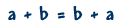Commutative property of multiplication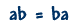Associative property of addition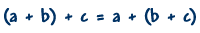Associative property of muliplication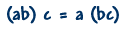Distributive property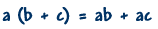The commutative property of addition says that we can add numbers in any order. You can remember the commutative property by thinking of the numbers "commuting," or changing places. The example shows us that "negative two plus positive four" is the same as "positive four plus negative two."

-2 + 4 = 4 + (-2)

Commutative property of multiplication
The commutative property of multiplication is very similar. It says that we can multiply numbers in any order we want without changing the result. The example shows us that "negative two times positive four" is the same as "positive four times negative two."

-2(4) = 4(-2)

The associative property of addition tells us that we can group numbers in a sum in any way we want and still get the same answer. You can remember the associative property by thinking of two numbers associating with each other, and then one leaves to associate with another number.

The example shows us that we can either add "negative two and positive four" together and then add that sum to positive three to get the final answer, or we can add "positive four and positive three" together first and then add that sum to negative two to get the final answer. The answer will be the same no matter which way we do it.

(-2 + 4) + 3 = -2 + (4 + 3)

Associative property of multiplication
The associative property of multiplication tells us that we can group numbers in a product in any way we want and still get the same answer.

The example shows us that we can either multiply "negative two and positive four" together and then multiply that product times positive three to get the final answer, or we can multiply "positive four and positive three" together first and then multiply that product times negative two to get the final answer. The answer will be the same no matter which way we do it.

-2(4) x 3 = -2(4 x 3)

Distributive property
The distributive property comes into play when an expression involving addition is then multiplied by something. It tells us that we can add first and then multiply, or multiply first and then add. Either way, the multiplication is "distributed" over all the terms inside the parentheses.

In the example, we can either add the numbers inside the parentheses first -- 4+3 -- and then multiply the result by -2; or, we can multiply the -2 and each term separately and then add the two products together. The answer is the same in both cases.

-2(4 + 3) = (-2 x 4) + (-2 x 3)

Watch out!

Subtraction is neither commutative nor associative.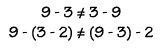Division is neither commutative nor associative.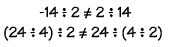Examples

1. We can add numbers in any order. (Commutative property of addition.)

-2 + 4 = 4 + -2

2. We can multiply numbers in any order. (Commutative property of multiplication)

-2 x 4 = 4 x-2

3. We can group numbers in a sum any way we want. (Associative property of addition.)

(-2 + 4) + 3 = -2 + (4 + 3)

4. We can group numbers in a product any way we want. (Associative property of multiplication)

(-2 x 4) x 3 = -2 x (4 x3)

5. With this type of expression, we can add first, then multiply,

OR

 Homework Help | Pre-Algebra | NumbersEmail this page to a friendSearch·  Place value·  Decimal numbers·  Estimating and     rounding·  Adding / subtracting     decimals·  Multiplying decimals·  Dividing decimals·  Percent·  Exponents·  Square roots·  Signed integers·  Adding and     subtracting integers·  Multiplying and     dividing integers·  Properties of integersFirst Glance In Depth Examples WorkoutProperties of integers